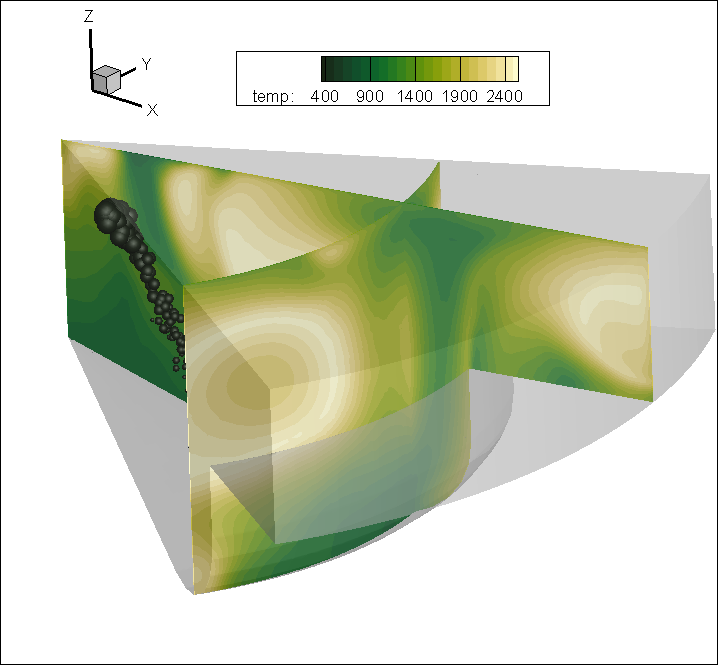# How do I create radial and theta slices?

## Radial slices

Compute the equation: {R} = sqrt(X**2 + Y**2) in the Data -> Alter -> Specify Equations dialog.  Turn on iso-surfaces in the Plot Sidebar. Then display an iso-surface of constant R.

## Theta slices

Compute the equation: {Theta} = atan2(X,Y) in the Data -> Alter -> Specify Equations dialog. Then display an iso-surface of constant Theta.  Note that due to a singularity at the origin you may get some strange shapes near zero.